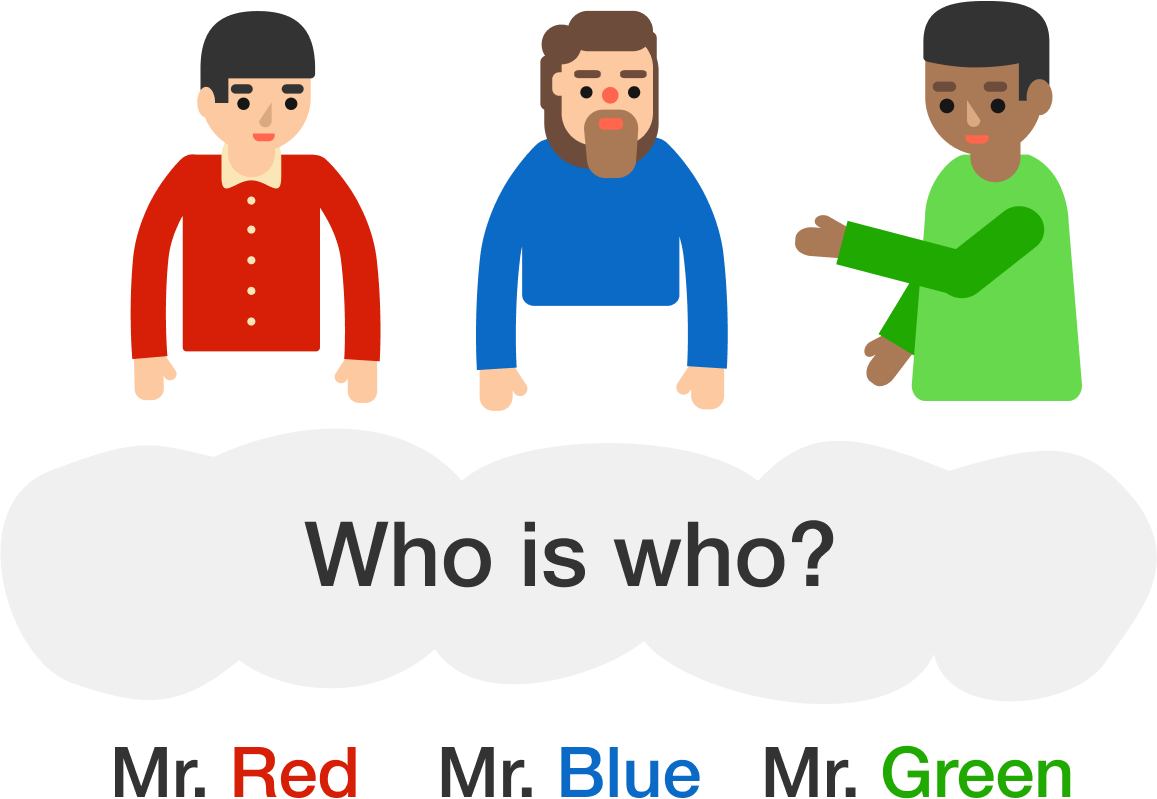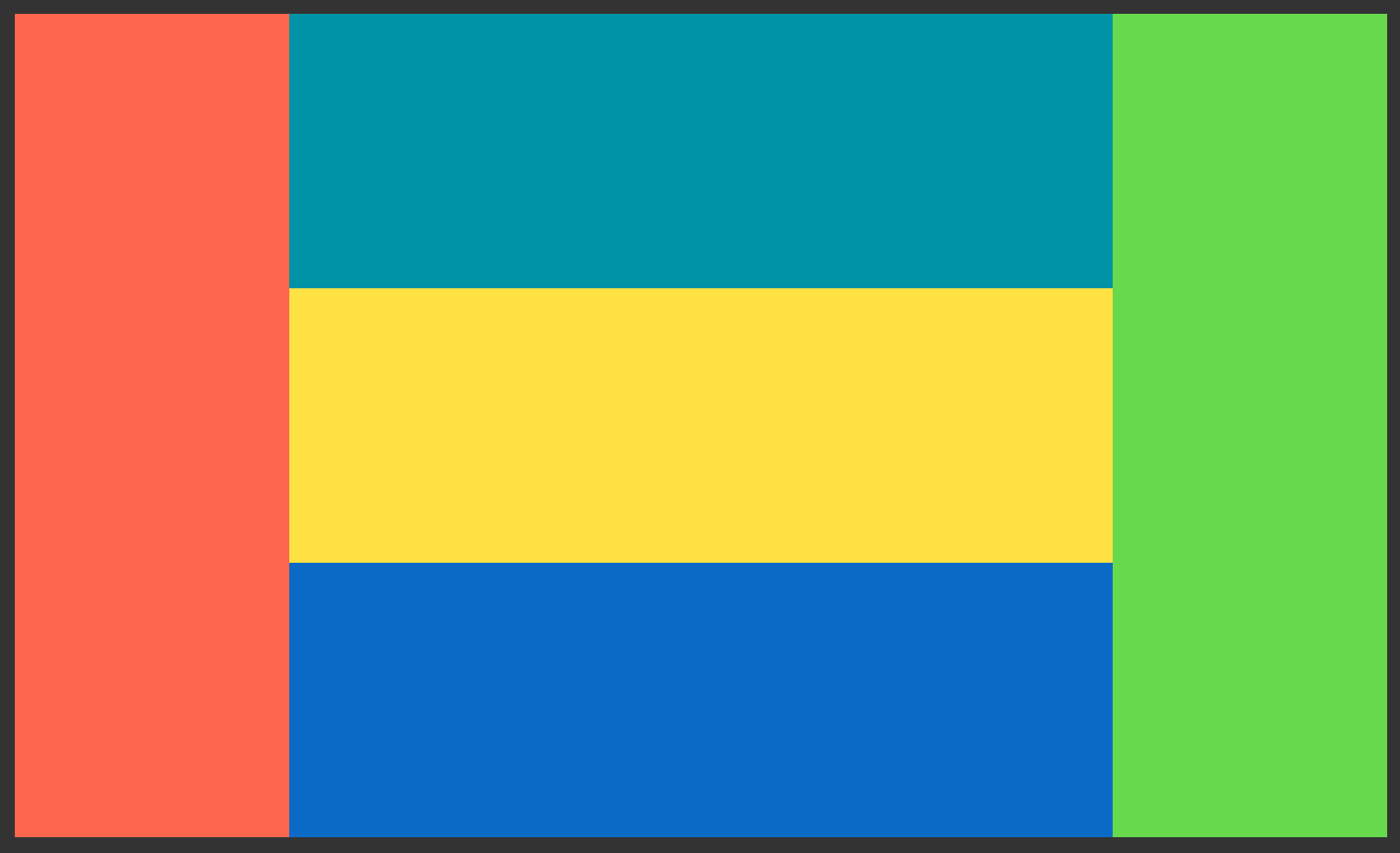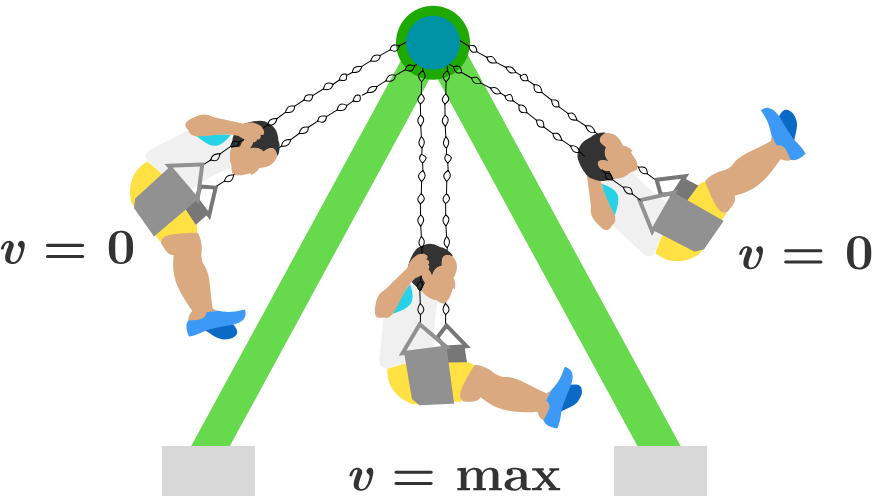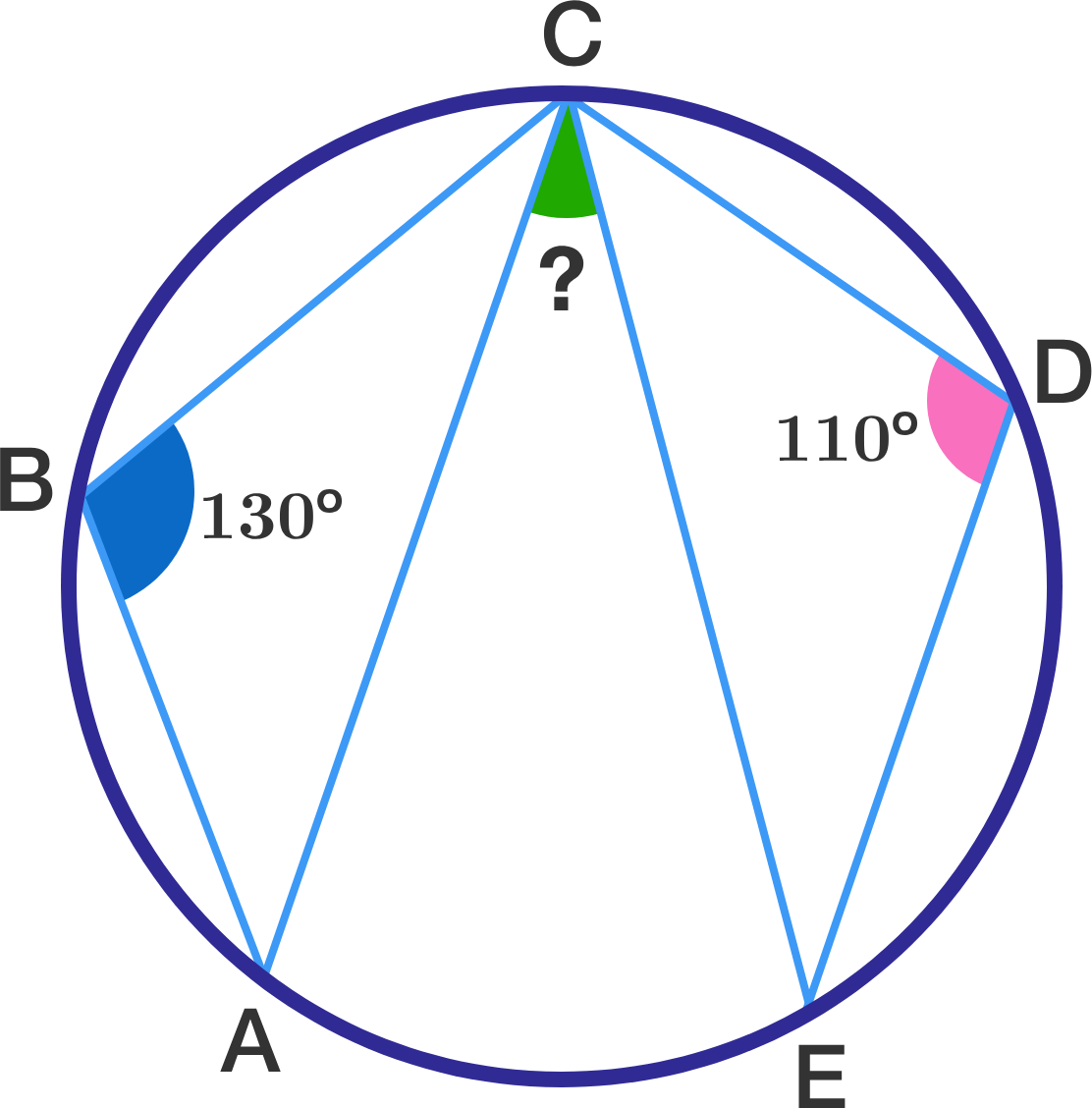# Problems of the Week

Contribute a problem

# 2017-03-13 Basic

$\LARGE {\color{#20A900}3}^{\color{#3D99F6}{2017}}-{\color{#20A900} 3}^{\color{#3D99F6}{2016}} = \, ?$Mr. Red, Mr. Blue, and Mr. Green are sitting at a table.
One man is wearing a red shirt, the second a blue shirt, and the third a green shirt.

Mr. Blue says, "Have you guys noticed how we're all wearing shirts of different colors from our names?"

The man in the green shirt replies, "Yes, Mr. Blue, I just noticed that!"

What is the color of the shirt that Mr. Blue is wearing?The big rectangle above is composed of 5 small identical rectangles. If the perimeter of the big rectangle is 80, then what is the area of a small rectangle?A little boy swings back and forth on the playground. At the highest point in his swing his speed is zero, and at his lowest point his speed is greatest.

Where in the trajectory will his acceleration be zero?

###### Caution: The acceleration is the rate of change of velocity and not the rate of change of speed.The points $A$, $B$, $C$, $D$, and $E$ are marked on the circumference of a circle in order such that $\angle ABC = 130^\circ$ and $\angle CDE = 110^\circ$.

Find the measure of the green angle, $\angle ACE$, in degrees.

Note: The diagram is not necessarily up to scale.

×